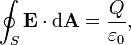Home » Electrostatics

# Category Archives: Electrostatics

## Problems from Electricity

1. The parallel plates of an air filled capacitor with area of area 1.1 x 10^8 m^2 . What must be the width of the plate be if the Capacitance is 1 Farad?
2. Four capacitor each of 2microfarad are connected in such a way that total capacitance is also 2microfarad. Show what combination gives this value. Also try to illustrate the diagram.

(Fari posted these)

1.Using the formula:

and substituting , C= 1 F, A = 1.1 x 108,  = 8.8 x 10-12,

we get  , d =9.68×10-4 metres## Is vacuum a Dielectric?

Is vacuum a Dielectric. If so how? please explain physically and mathematically

Vacuum cannot be considered as a dieletric.

A dielectric is defined as an insulating material which can be polarized by applying electric field. When a dielectric is placed in an electric field, electric charges do not flow through the material, but only slightly shift from their average equilibrium positions causing dielectric polarization.

Nothing like that will happen in the case of vacuum.

But in theory, if necessary, vacuum can be considered as a dielectric medium of dielectric constant unity.

## How does dielectric constant depend on nature of medium?

How does dielectric constant depend on nature of medium?
Does this have any relation with free electrons and force between molecules?

Dielectric Constant, also called relative permitivity of a medium depends on the electric susceptibility of it, the ease with electric flux lines can pass through it.

The susceptibility depend on molecular polarization, the electric dipole moment acquired per unit volume in an external electric field.

So, the thing to note is that, dielectric constant is a physical quantity measured as a comparison of the strength of electric field in vacuum to that inside the material.Dielectric constant can be defined in terms of electrostatic force

Dielectric Constant can also be defined in terms of capacitance.

## Gauss Theorem – Electrostatics

“WHAT IS THE  MAGNITUDE OF ELECTRIC  FIELD IN THE  GAUSSIAN SURFACE OF  A CUBE,AT ITS FACE ,AT ITS CENTRE, AT ITS CORNERS OR AT ANY OTHER POINT INSIDE THE CUBE. ALSO TELL ME THE THE WAY TO KNOW IT.”

Ans:

According to Gauss Theorem, the total electric flux through a closed surface,The Gaussian surface is an imaginary surface. So, for calculating the electric field at a point using Gauss theorem, we have to imagine a Gaussian surface symmetric with the given charge distribution.

I have assumed that there is a point charge Q at the centre of the cube.

At its face (at a point on the face on the line radially outwards from the point charge at the centre of the cube and perpendicular to the face)

The distance is equal to half the length of side of the cube (Say L).

On the corner,

Calculate the distance from the centre of the cube to its corner and replace (L/2) in the above equation with that distance.

### Hits so far @ AskPhysics

• 2,182,326 hits

### CBSE Exam Count Down

AISSCE / AISSE 2020February 26, 2020
38 days to go.